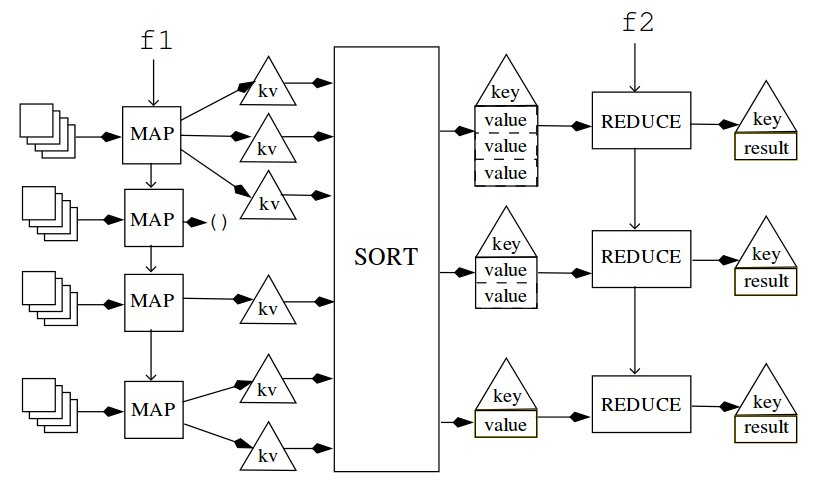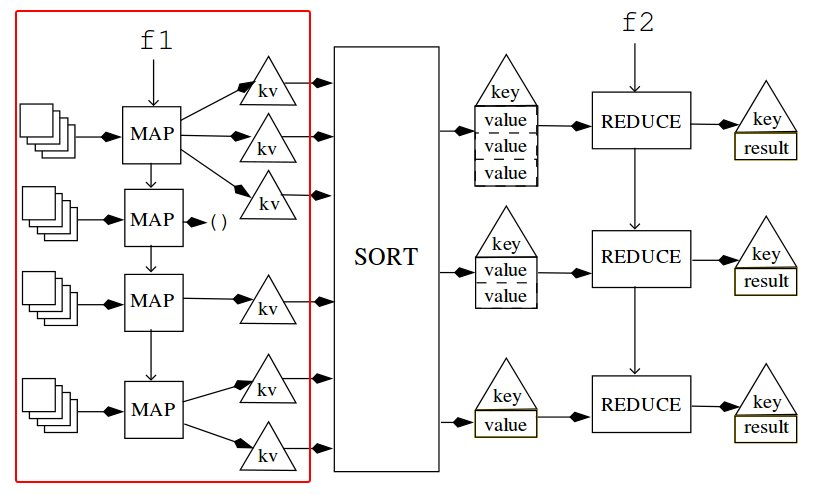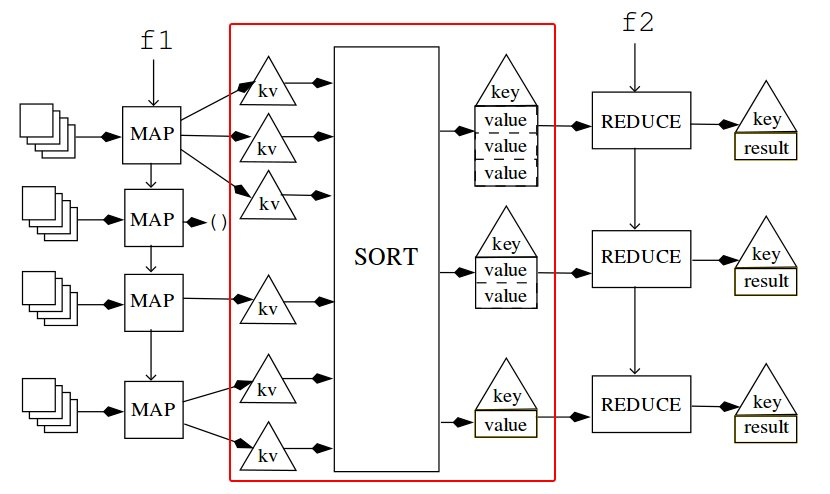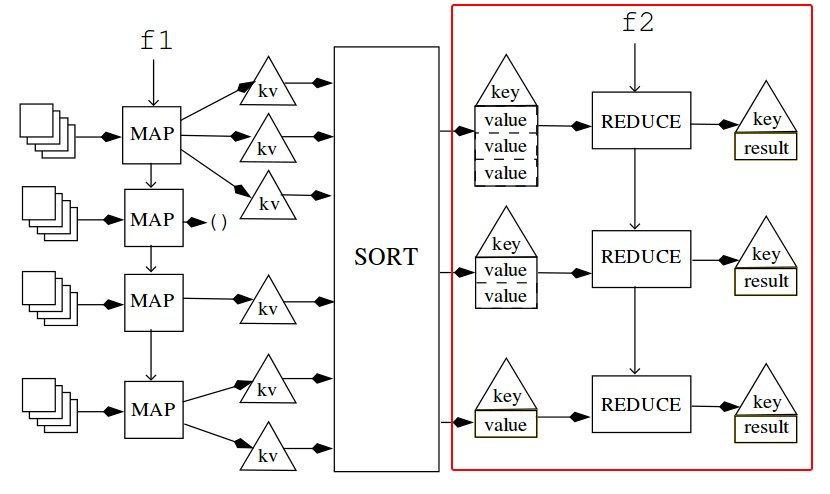# Mapreduce

### Introduction to Mapreduce

In this section, we will revisit higher order functions from Unit 2 (map and accumulate) and combine it with parallelism which enables us to process a huge amount of data efficiently.

### Background to Mapreduce

Engineers in Google noticed that a majority of their computations could be broken down into a map of some function over data, followed by an accumulate (also known as reduce, hence the name) afterwards. The result is a library procedure named mapreduce that takes two functions as arguments; one that acts as a mapper and another that acts as a reducer. It accepts a large chunk of data, divide them into smaller parts, apply the mapper to the smaller sized data and combines the results with a reducer. Mapreduce handles everything related to parallelism, and we only have to provide the 2 functions.

Although this may seem like what mapreduce is doing, this is not mapreduce:

(define (mapreduce mapper reducer base-case data)
(accumulate reducer base-case (map mapper data)))


Why is that not mapreduce? Because it doesn't handle dividing the data, applying the mapper parallelism and sorting them before reducing them. What it gets right though is that whoever wants to use mapreduce only needs to pass four arguments: mapper, reducer, base-case and the datawe want to process to the mapreduce function.

If you are interested, here is an paper written by the Google employees who came up with mapreduce. It is not required, but it is pretty readable and interesting. The old lecture notes also have a good explanation, and you should read those if you feel like you don't understand mapreduce.

### Breakdown of Mapreduce1. Map the mapper to the smaller data (done in parallel). This involves selecting parts of the input we want to process and "attaching" a key to each results
2. Sort the result to 'buckets' based on their keys
3. Each 'bucket' is passed to a reducer that accumulates the values

We will look at each of these steps into more detail in subsequence sections.

Throughout this lesson, we will use the example of searching through a text file and find the frequency of the words.

This will act as our test files:

>(define song1   '( ((please please me) i saw her standing there)

>(define song2   '( ((with the beatles) it wont be long)
((with the beatles) all ive got to do)
((with the beatles) all my loving)))

>(define song3   '( ((a hard days night) a hard days night)
((a hard days night) i should have known better)
((a hard days night) if i fell)))

>(define all-songs (append song1 song2 song3))
((with the beatles) it wont be long)
((with the beatles) all i have got to do)
((with the beatles) all my loving)
((a hard days night) a hard days night)
((a hard days night) i should have known better)
((a hard days night) if i fell) )


Note that every line is 'tagged' with their titles. We recommend keeping this tab open somewhere so that you know what our input looks like.

You can get this data and the other functions of our implementation of mapreduce here. Note that this implementation does not involve parallelism.

### Mapper(map mapper data)

• Input: (smaller) A key-value pair
• Output: list of key-value pairs

A mapper is a function that accepts data (as a key-value pair), and returns a list of key-value pairs. A list of key-value pairs is the same as associative lists (also known as a-lists) that we played with in lesson 9. The keys are used to keep track of where the data is from; this is important for the parallelization. Note that the key of the input is not neccessarily the same as the key(s) that the mapper outputs. This will be our ADT for kv-pair:

    (define make-kv-pair cons)
(define kv-key car)
(define kv-value cdr)


If we look at the first item of our sample input, the result is the following :

 >(kv-key '((please please me) i saw her standing there))
(i saw her standing there)


Why do we output a list of key-value pairs instead of a single key-value pair? Here are some reasons:

• no key-value: There will be cases where our data does not contain a key that is of an interest to us. For example imagine a case where you want to count number of vowels in a word, and you encounter the word 'fly'. We would return the empty list in this case.
• multiple key-value: There will be cases where our data corresponds to 2 or more keys that we want to produce. This is applicable to our song lyrics example (shown below)

### Extended Example: Word-Count

Here is the definition of a mapper for our example.

>(define (mapper input-kv-pair)
(map (lambda (wd) (make-kv-pair wd 1)) (kv-value input-kv-pair)))

((i . 1) (saw . 1) (her . 1) (standing . 1) (there . 1))



What does our mapper do? It accepts a key-value pair (its key is the song title, its value is a line from the song). For every word in the lines, use the word as the new key, and pair that with the value 1. Note that even if a word appears twice in the line, like '(please please me), it outputs (please . 1) twice and NOT (please . 2). That is fine, because here we are only starting the count for each at 1. We add them up later.

### sort-into-bucketsBefore we actually get into the reducer, there is an intermediary step that sorts the keys, and group the same keys together. Fortunately, we can take advantage of abstraction and use the function **sort-into-buckets** to sort them into 'buckets'for us. key-value pairs with the same keys are grouped under the same 'bucket'. This is the result of calling the mapper from the previous step:

>(map mapper all-songs)
( ((i . 1) (saw . 1) (her . 1) (standing . 1) (there . 1))
((misery . 1))
((it . 1) (wont . 1) (be . 1) (long . 1))
((all . 1) (i . 1) (have . 1) (got . 1) (to . 1) (do . 1))
((all . 1) (my . 1) (loving . 1))
((a . 1) (hard . 1) (days . 1) (night . 1))
((i . 1) (should . 1) (have . 1) (known . 1) (better . 1))
((if . 1) (i . 1) (fell . 1)) )


Calling sort-into-buckets results inthe following:

>(sort-into-buckets (map mapper all-songs))

'( ((i . 1) (i . 1) (i . 1) (i . 1))
((saw . 1))
((her . 1))
((standing . 1))
. . .
((all . 1) (all . 1))
((have . 1) (have . 1))
. . .
((if . 1))
((fell . 1)) )


Some parts of the result is ommited to keep it short. Note how the keys and values are organized here. The result is a list of buckets, where a bucket is a list of kv-pair with the same keys. ((i . 1) (i . 1) (i . 1) (i . 1)) is an example of a bucket, where each kv-pair has the key 'i'.

### Reducer• Input: two "values"
• Output: a value

A reducer accepts two values(without the keys), and outputs a single value. This will be the output associated with that particular key.

### Extended Example: Word-Count

Here is the definition for our reducer

(define (reducer num other-num)
(+ num other-num))


### Reducing One Bucket

Note that our reducer accepts two values while the result of our previous step (sort-into-buckets) is a list of buckets (where a bucket is a list of key- value pairs). Let's look at how we can use our reducer to a single key- value. Let us use our first list of key-value:

((i . 1) (i . 1) (i . 1) (i. 1) (i . 1))


> (accumulate reducer 0 (map kv-value '((i . 1) (i . 1) (i . 1) (i . 1) (i . 1))))

this simplifies to:

> (accumulate reducer 0 '(1 1 1 1 1))

5

Before we call accumulate, we have to obtain the values from the list of kv- pairs by using map. Note that the result of calling accumulate is a single value (the 5) associated with the key (which is 'i' in this case). Because our end result needs to be a kv-pair, we have to return (i . 5) in the end. The expression then becomes:

(make-kv-pair (kv-key '(i . 1))
(accumulate reducer 0 (map kv-value '((i . 1) (i . 1)
(i . 1) (i . 1) (i . 1)))))


We can generalize the expression above into any other "bucket" besides '((i . 1) (i . 1) (i . 1) (i . 1) (i . 1)).

(define (reduce-bucket reducer base-value bucket)
(make-kv-pair   (kv-key (car bucket))
(accumulate reducer base-value (map kv-value bucket))))


### Reducing a List of Buckets

The procedure reduce-bucket above reduces one bucket. Our result from the previous step, (sort-into-buckets (map mapper data)) is a list of buckets. To reduce a list of buckets, we can use map again. We are going to define the function group-reduce that does exactly that.

(define (groupreduce reducer base-case buckets)
(map (lambda (bucket) (reduce-bucket reducer base-case bucket))
buckets))


If we use what we have so far, it evaluates into the following:

>(groupreduce reducer 0 (sort-into-buckets (map mapper all-songs)))
( (i . 4) (saw . 1) (her . 1)
. . .
(misery . 1) (please . 2) (me . 1)
. . .
(all . 2) (have . 2))


Some values are omitted for conciseness. The final result is again, a list of kv-pairs: the key is the word, the value is how many times those words appear in our data. We have just used mapreduce to construct word counts for words in our data!

This is the final (hand-wavy, approximated) definition of our mapreduce:

(define (mapreduce mapper reducer base-case data)
(groupreduce reducer base-case (sort-into-buckets (map mapper data))))


Why is it not the actual mapreduce? The actual one will involve mapping and reducing our kv-pairs parallely and we have to take into account of concurrency issues. The definition above captures the major parts that we are concerned with.

### Practice with Mapreduce

Writing a mapreduce function is all about defining your mapper and reducer. We have series of different scenarios and want you guys to define the corresponding mapper, reducer and at the end the call to mapreduce.

Remember:

• The input to your mapper is a key-value-pair and it outputs a list of key-value pairs.
• The input to your reducer are two values and it outputs one value

### Chaining Mapreduce

As hinted before, since mapreduce takes as an input a list of key-value pairs and outputs a list of key-value pairs, it is possible to chain mapreduce together. It would look something like this: (mapreduce some-mapper some- reducer some-base-case (mapreduce another-mapper another-reducer another-base- case actual-input)). Note that the keys and values for the first mapreduce may be totally different from your second mapreduce.

### Most Frequent Word

Let's write another mapreduce function (We're not chaining yet). This time, our input has a key of 'words' and the value are numbers, representing how many times they appeared in a document. We want our output to be a list of a single key-value pair where just like the input, our key is a word and our value is a number such that it is the highest number encountered.

Note: our solution isn't ideal, and it's a little contrived. It doesn't take advantage of the parallelism that mapreduce offers.

>(define x (list (make-kv-pair her 1) (make-kv-pair i 4) (make-kv-pair saw 1))
>(most-frequent x) ; i appears the most
((i . 4))


### Now We're Chaining

Our function above works, if we pass the pairs with the key as a word, and value as a number. In real life, we might not have direct access to the word counts of each word; we have to process that from the original document.

Write the function real-most-frequent that accepts a list of key-value pairs where the key is the name of the file, and the values are lines from that file (just like our all-songs example) . Our output is again, a list of single key-value pair. You may want to reuse any functions we have defined so far in the lesson.

>(real-most-frequent all-songs)
((i . 4))


The reader does not contain MapReduce exercises. If you want to get more practices, there are MapReduce questions on the Lesson 14 discussion.

14 - Concurrency and MapReduce